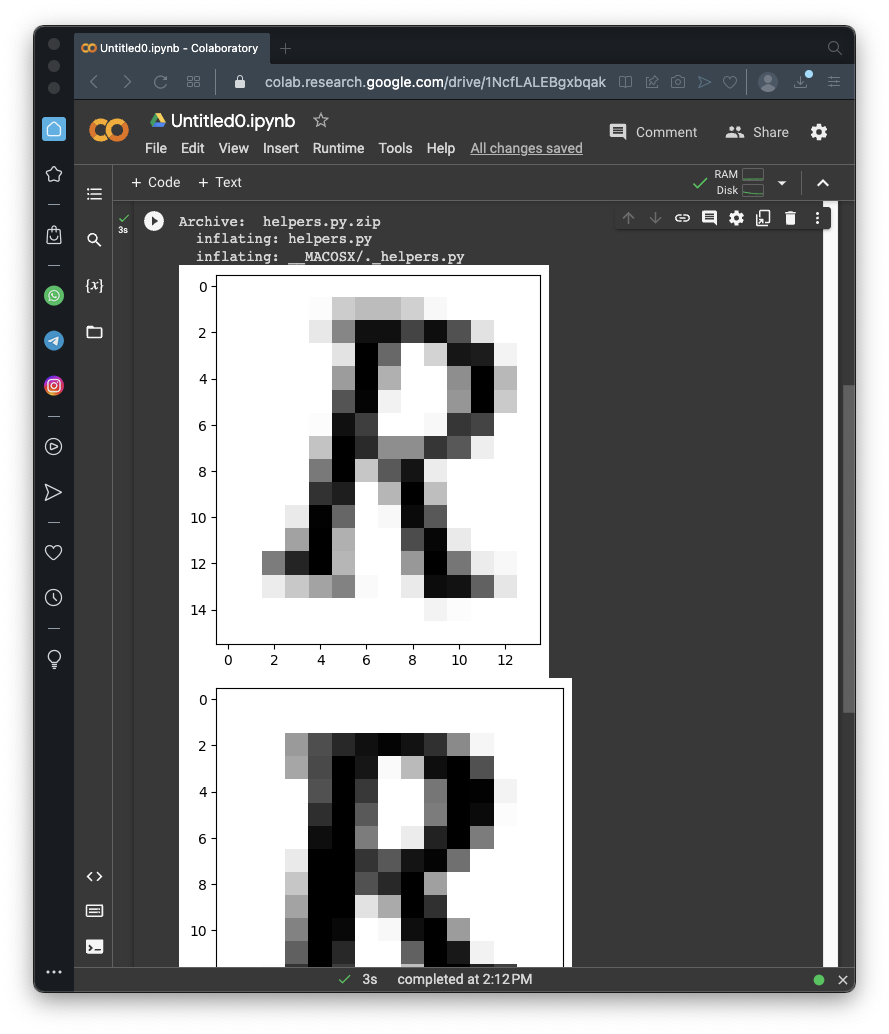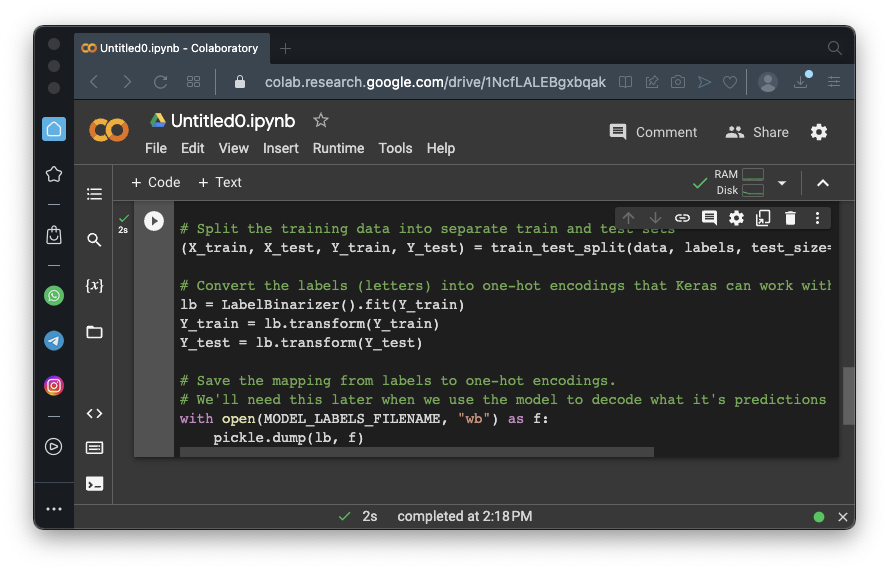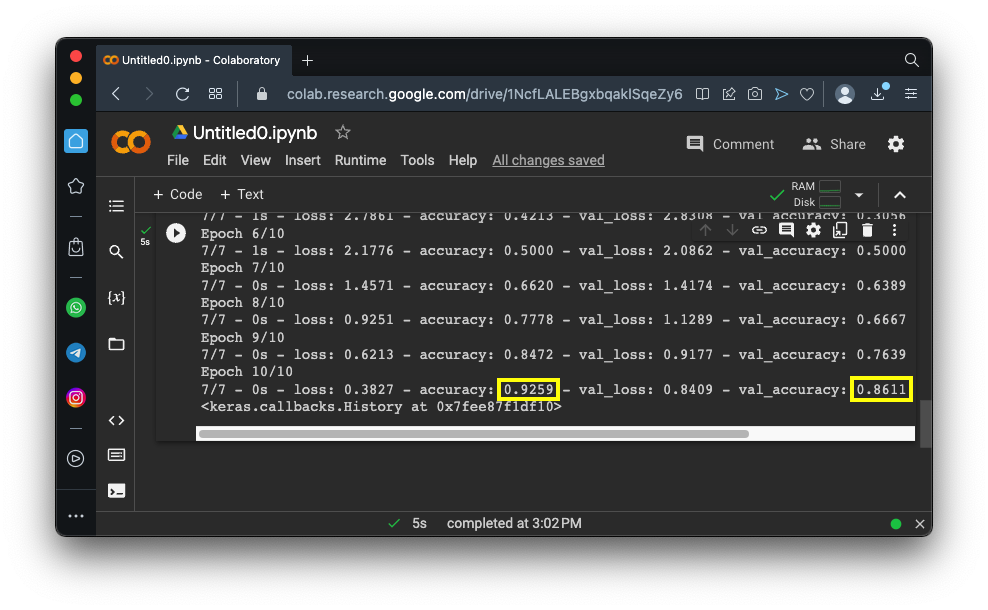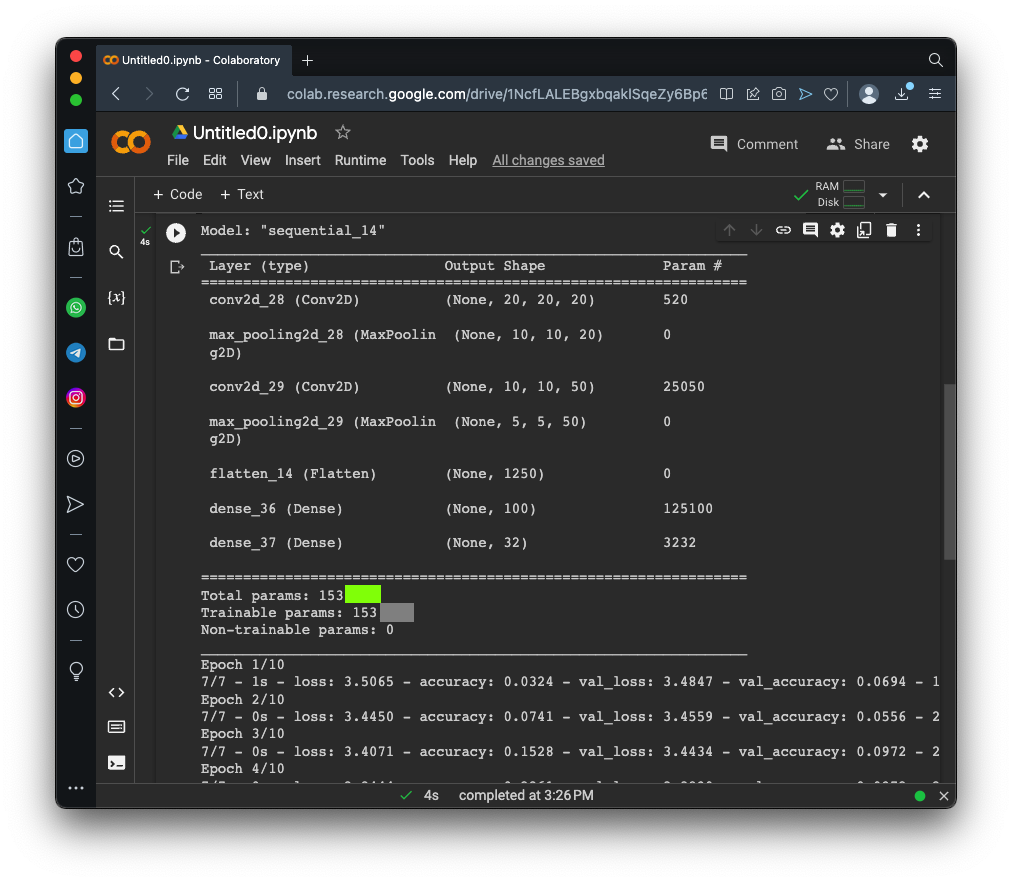# ML 102: Breaking a CAPTCHA (10 pts)

## What You Need

• A Web browser

## Purpose

To practice making simple machine learning code in Python.

In a browser, go to

From the menu, click File, "New notebook".

```!wget https://samsclass.info/129S/proj/content2.zip !unzip content2.zip !wget https://samsclass.info/129S/proj/helpers.py.zip !unzip helpers.py.zip import matplotlib.pyplot as plt import imageio as iio image = iio.v2.imread("content2/extracted_letter_images/R/001099.png") plt.imshow(image, cmap='gray') plt.show() image = iio.v2.imread("content2/extracted_letter_images/R/000387.png") plt.imshow(image, cmap='gray') plt.show() ```
As shown below, the images download and the first two are displayed. These are various deformed versions of letters and numbers.## Preparing the Images

This process reduces all the images to 20x20 grayscale images and saves the labels to mark the correct letter for each one.

Delete all the code.

Enter the code below:

```import cv2 import pickle import os.path import numpy as np from imutils import paths from sklearn.preprocessing import LabelBinarizer from sklearn.model_selection import train_test_split from helpers import resize_to_fit LETTER_IMAGES_FOLDER = "content2/extracted_letter_images" MODEL_FILENAME = "captcha_model.hdf5" MODEL_LABELS_FILENAME = "model_labels.dat" # initialize the data and labels data = [] labels = [] # loop over the input images for image_file in paths.list_images(LETTER_IMAGES_FOLDER): # Load the image and convert it to grayscale image = cv2.imread(image_file) image = cv2.cvtColor(image, cv2.COLOR_BGR2GRAY) # Resize the letter so it fits in a 20x20 pixel box image = resize_to_fit(image, 20, 20) # Add a third channel dimension to the image to make Keras happy image = np.expand_dims(image, axis=2) # Grab the name of the letter based on the folder it was in label = image_file.split(os.path.sep)[-2] # Add the letter image and it's label to our training data data.append(image) labels.append(label) # scale the raw pixel intensities to the range [0, 1] (this improves training) data = np.array(data, dtype="float") / 255.0 labels = np.array(labels) # Split the training data into separate train and test sets (X_train, X_test, Y_train, Y_test) = train_test_split(data, labels, test_size=0.25, random_state=0) # Convert the labels (letters) into one-hot encodings that Keras can work with lb = LabelBinarizer().fit(Y_train) Y_train = lb.transform(Y_train) Y_test = lb.transform(Y_test) # Save the mapping from labels to one-hot encodings. # We'll need this later when we use the model to decode what it's predictions mean with open(MODEL_LABELS_FILENAME, "wb") as f: pickle.dump(lb, f) ```
Click the Run button.

The code runs, but doesn't print out any output. You can see a "completed" message at the bottom of the Colab window, as shown below.## Creating a Model and Training

Delete all the code.

Enter the code below:

```from keras.models import Sequential from keras.layers import Conv2D, MaxPooling2D from keras.layers import Flatten, Dense model = Sequential() # First convolutional layer with max pooling model.add(Conv2D(20, (5, 5), padding="same", input_shape=(20, 20, 1), activation="relu")) model.add(MaxPooling2D(pool_size=(2, 2), strides=(2, 2))) # Second convolutional layer with max pooling model.add(Conv2D(50, (5, 5), padding="same", activation="relu")) model.add(MaxPooling2D(pool_size=(2, 2), strides=(2, 2))) # Hidden layer with 500 nodes model.add(Flatten()) model.add(Dense(500, activation="relu")) # Output layer with 32 nodes (one for each possible letter/number we predict) model.add(Dense(32, activation="softmax")) # Ask Keras to build the TensorFlow model behind the scenes model.compile(loss="categorical_crossentropy", optimizer="adam", metrics=["accuracy"]) model.summary() model.fit(X_train, Y_train, validation_data=(X_test, Y_test), batch_size=32, epochs=10, verbose=2) ```
Click the Run button.

As shown below, the model attains 93% accuracy on the training data and 86% on the test data.## Flag ML 102.1: Model Summary (10 pts)

Change the Dense line to the line shown below, wso there are only 100 hidden nodes:
```model.add(Dense(100, activation="relu")) ```
Run this model.

The flag is covered by a green rectangle in the image below.## Sources

AI and Machine Learning for Coders: A Programmer's Guide to Artificial Intelligence
Breaking captchas with deep learning, Keras, and TensorFlow

Posted 4-10-23
Video updated 4-20-23
"extra" removed from points 9-16-23
keras import statements fixed 9-16-23